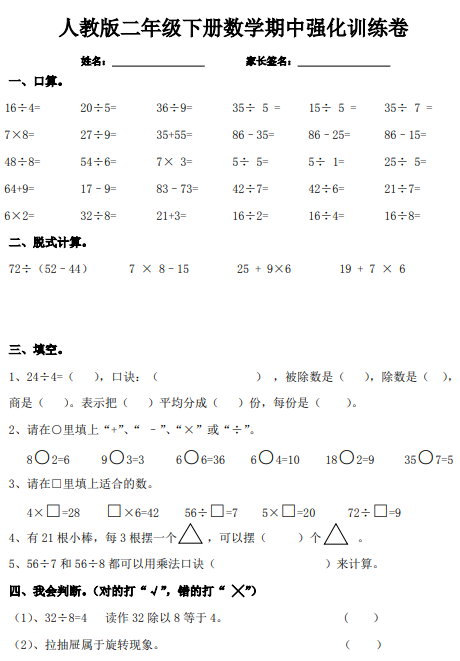16÷4= 20÷5= 36÷9= 35÷ 5 = 15÷ 5 = 35÷ 7 =
7×8= 27÷9= 35+55= 86–35= 86–25= 86–15=
48÷8= 54÷6= 7× 3= 5÷ 5= 5÷ 1= 25÷ 5=

【PDF文档2页】最新人教版小学二年级下册数学期中检测题 (第十份)A4电子版资料_可直接打印_会员免费下载##### 评论信息